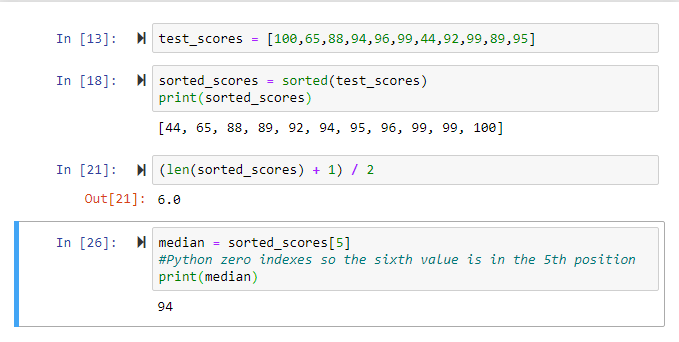In this tutorial, we'll cover the cental tendency statistic, the median. The median is the middle value in a dataset when ordered from largest to smallest or smallest to largest.

Now that we have learned about the mean, let’s learn another central tendency statistic: median. The median refers to the middle value in a dataset, but the dataset must be ordered either smallest to largest or largest to smallest. Depending on the size of your dataset, the median can either be a quick hand calculation or a very tedious task that is open to many mistakes. Furthermore, if the dataset contains an odd number of values calculating is more straight forward than if it contains an even number of values because you have to cross off values and then calculate the average between the two leftover numbers (do not worry so much about this, thanks to an easy formula we will introduce).

### The Use for the Median

Before learning how to calculate the median, let’s discuss why we would ever want to solve for the median. In school and in everyday life, people prefer to use averages over the median, so why take time and learn about the median? We only really use the median in reference to “income” and “house prices”, but why does this phenomenon of only using the median in rare occasion exist. I only think the reason we do not use the median is that it can be a pain to solve. However, I think the median is actually a better measurement of data than the mean, at least in a majority of cases. Especially, when Python does most of the heavy “lifting” for you, therefore I would always prefer to use the median as a more effective summary metric.

The median does not get affected by outliers of data and is virtually impossible to be skewed. To find the median of a small dataset, the quickest method by hand is to cross off one number on each side until you get to the middle number. Let’s look at a quick example, there is a class of 11 students and their grades are as follows: 44, 65, 88, 89, 92, 94, 95, 96, 99, 99, 100. The class mean is an 87 while the median which we will solve by the cross-out method (44, 65, 88, 89, 92, 94, 95, 96, 99, 99, 100), so the median is 94. Now let’s take a step back and look at the grades again and now ask yourself which measurement is more reflective of the overall class success on the exam? The median is because 9 out of 11 students performed really well on the exam and only two did poorly, but the mean shows that the class all did well but not great on the exam, but the median shows the true summary of the data.

What if a dataset is large and ordering and crossing out is extremely time-consuming and leaving the ability of human error to take over? A good shorthand trick to calculate the median is to follow these three steps. The first step is to order the list in smallest to largest or vice versa. The second step is to count how many data points are in your set, so if we are using the test scores example from the above that number will be 11. The third step is using the formula: (number of data points + 1) / 2. This formula does not give you the value of the mean but rather the position in the list. So, going back to the grades example, the formula would produce a value of six and the grade in the 6th position of the list is 94. Keep in mind, if the answer is a decimal, such as 6.5, then take the average between the value of data in the 6th and 7th positions.

### Median in Python

So now that we know how to solve and why we would want to solve for the median, let’s look at how to program to get the median in Python. It is always important to master the mathematical concept by hand since Python does the calculation “behind the scenes”. Below, I am going to show how to get the median in vanilla Python with a data type such as a list. The second example which will be covered in a couple of articles will be much simpler but can only be used if you imported pandas and your data is organized in a dataframe. If these last two sentences confuse you, do not worry just stop reading and sign up for one of these awesome Python Courses or Data Science Classes that are offered both in-person in NYC or live online.

### Median Tutorial

• Step 1: Create a variable named test_scores and populate it with a list of individual test scores.

• Step 2: Create a variable named sorted_scores and set it equal to sorted(test_scores), the sorted function puts the test_scores in order from smallest to largest.

• Step 3: Use the len attribute on sorted_scores to get the number of values in the list (same as we did with the mean) and added one to it and divided it by 2 (this was the formula highlighted above).

• Step 4: Take the answer you get from Step 3 and write sorted_scores, this will give us the score in the 6th position. Python uses zero indexing, so the fifth position is the sixth element since we start counting from zero. Finally, set that value equal to the median and print median.